Calibration Function (EU Function)

Measured values are read as a voltage value. If the reference value of the signal under measurement (acceleration, pressure, sound, etc.) is defined, it can be read as a physical quantity by calibrating the voltage value to the reference value.

Example 1:
When the input of the acceleration pickup is 1m/s2 and the output 100mV, the physical quantity is 10.0EU/V (10 times the obtained voltage value) and the unit is m/s2.

Example 2:
When calibrating a sound level meter using a microphone and a sound calibrator, the overall value (in dB) is used as the output sound pressure level of the sound calibrator by means of the power spectrum data.

Cepstrum

The cepstrum is obtained by the Fourier transform of the logarithmic value of the power spectrum obtained by the Fourier transform. The horizontal axis of the cepstrum is assigned a time-dimensional value referred to as quefrency.

If a signal input to a certain system has a periodicity and the period is long, the period appears as a linear cepstrum in the long quefrency portion, and can be extracted as a fundamental period. The information representing the transfer characteristic of the system is concentrated in the short quefrency portion. The envelope of the power spectrum is obtained by performing the inverse Fourier transform of this portion. The liftered envelope is specific to the system and does not depend on the spectrum of the input signal.

Applications of the cepstrum include extraction of the fundamental frequency and spectrum envelope from sound wave, organism wave, and so on.

Channel Delay Function

In measurement of the transfer function of a mechanical system, if the system has a long signal propagation time causing temporal shift (delay) between the input signal and the output signal of the system, the transfer function cannot be measured accurately.

If there is temporal shift between channels, the delay function is used to correct the timing by delaying the sampling start timing of the slave channel with respect to the sampling start timing of the master channel.

Coherence Blanking Function

A small value of the coherence function HREF_COH, γ2 (f), between two channels under measurement indicates that the measurement result is not accurate. The coherence blank function does not display such inaccurate components but displays only components with large γ2 (f). The value of γ2 (f) can be set to any value. The transfer function is not displayed at frequencies when γ2 is equal to or less than the specified value

Coherence Function

The coherence function γ2 indicates the degree of the correlation between the input and output of a system. It gives a value from 0 to 1 for each frequency.

When γ2 (f) is 1, it indicates that at frequency f all outputs of the system are due to the input. When γ2 (f) is 0, it indicates that at frequency f the output of the system is completely independent of the input.

When the following condition is met,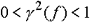presence of noise occurred inside the system or non-linearity or time delay of the system can be assumed.

?2 is obtained by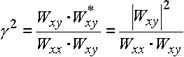where Wxy is the cross spectrum and Wxx and Wyy are the power spectrum of x and y, respectively. The coherence function, γ2, is the square of the absolute value of the cross spectrum divided by each power spectrum of the input and output of the system.

The coherence function, in its nature, is not meaningful unless averaging is performed. When measuring the coherence function, be sure to perform averaging.

Coherence Output Power

The product of the coherence function and the auto power spectrum of the output signal is referred to as the coherent output power (C.O.P.).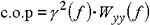The C.O.P represents only the auto power spectrum caused by the input under measurement out of the auto power spectrum of the output.

The coincident quadrature diagram is a diagram which plots the real part and imaginary part of the frequency response function separately on the frequency axis and displays them with vertical arrangement. This diagram is used for estimation of inherent vibration, etc.

It is plotting the real and imaginary parts of the frequency response function separately against the frequency axis and displays them side by side on the top and bottom. It can be used for estimating natural frequencies, for example.

Correlation Function

There are two types of correlation functions: auto-correlation function and cross-correlation function.

Crest Factor

The crest factor is defined as the ratio of the peak value to the RMS value (peak value/RMS value). The crest factor of a DC signal is 1 and that of a sine wave is √2=1.414.

As for the peak value and RMS value, for example, the vibration value changes relatively with the bearing size. (With large bearings, the RMS value of vibration is large and the peak value, under abnormal condition, becomes larger.) Since the crest factor denotes the ratio of the peak value to the RMS value, the vibration value is not affected by the bearing size, allowing accurate judgment of the extent of flaws and other abnormal conditions. If the value of the measured crest factor is large, a large extent of abnormal condition is judged.

Cross-Correlation Function

There are two types of correlation functions: auto-correlation function and cross-correlation function.

The cross-correlation function is a function of delay amount τ when the waveform of one of two waveforms is delayed by τ. It is defined by the following expression: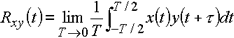The cross-correlation function is used for measurement of correlation and time delay between two signals. If the two signals are different completely, the cross-correlation function approaches zero irrespective of τ. If the two signals correspond to the input and output of a certain system, the cross-correlation function is used to estimate the delay time within the system, detect signals embedded in external noise, and estimate the signal propagation path.

With the FFT analyzer, the cross-correlation function is obtained by the inverse Fourier transform of the cross spectrum.

Cross Spectrum

When the Fourier transform of two signals x (t) and y (t) is X (f) and Y (f) respectively and the complex conjugate of X (f) is X*(f), the cross spectrum, Wxy (f), is defined by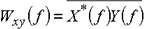The cross spectrum is given by obtaining the product of the same frequency component of the Fourier spectrum of two signals and then averaging the result. The X axis is represented by the frequency and the Y axis by V2. A large value of the cross spectrum at a certain frequency means that there is intense correlation between the frequency components of the two signals at the frequency and that the magnitudes of these components are large. The cross spectrum is used to calculate the cross-correlation function, transfer function, and coherence function.

Curve Fitting

In dynamic characteristic measurement of machines and other structures, the transfer function of the system is usually obtained by applying an impulse to the structure with a hammer and performing the FFT operation for the impulse response obtained.
However, the FFT-based transfer function is discrete data with limited regular-interval frequency resolution and therefore has a problem that there are very few measuring points near the characteristic frequency at which the function changes abruptly.  Therefore, the Nyquist diagram obtained from the transfer function does not provide the ideal circle tracing. To obtain correct peak values, characteristic frequency, and other modal parameters, curve fitting, which interpolates the regular-interval data during calculation, is necessary.

With the curve fitting method, an analytical expression of the transfer function is assumed and, by setting the characteristic frequency, damping factor, vibration mode, and other modal parameters to an appropriate value, the measured transfer function is approximated to the transfer function of the model.  This method determines theoretically the dynamic response of a structure in modal analysis.

In actual curve fitting, multiple points are plotted on the Nyquist line using the real part and imaginary part of the complex transfer function of the discrete system which is obtained as a result of measurement.  Then, a theoretical Nyquist diagram with the minimum error to these points is calculated, the transfer function is obtained from it, then the calculated transfer function is fit to the measured transfer function.

The following two methods are mainly used for curve fitting.  If the peaks of vibration mode are separated and do not have any mutual influence, SDOF (Single-Degree-Of-Freedom) curve fitting is used.  Conversely, if the adjacent vibration modes overlap each other, it is necessary to take the influence of many vibration modes into consideration, and a calculation algorithm is required which applies a number of modal parameters representing the transfer function analytically to the measured transfer function at the same time.  This method is referred to as MDOF (Multi-Degree-Of-Freedom) curve fitting.